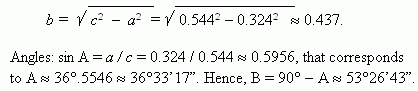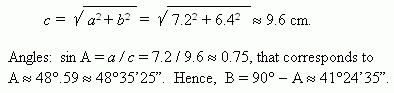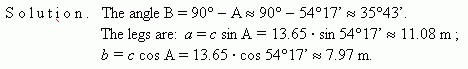# Solving of right-angled triangles

Solving of right-angled triangles:
by two sides, by a side and an acute angle.

1. By two sides. If two sides of a right-angled triangle are given, then the third side can becalculated by the Pythagorean theorem ( see the paragraph of the same name in the section "Triangle" of the part "Geometry" ). Acute angles are determined by one of the three firstformulas for trigonometric functions, depending on the fact what sides are known. Forinstance, if legs a and b are given, then angle A is determined by the formula:

tan A = a / b .

 E x a m p l e  1. A leg a = 0.324, a hypotenuse c = 0.544. Find the second leg b and the angles A and B. S o l u t i o n . The leg b is equal:E x a m p l e  2. Two legs are given: a = 7.2 cm, b = 6.4 cm. Find a hypotenuse and the angles A and B. S o l u t i o n . The hypotenuse c is equal:2. By a side and an acute angle. If one acute angle A is given, then another acute angle B is found as: B = 90° – A . Sides are found by formulas of trigonometric functions, rewritten as:

a = c sin A , b = c cos A , a = b tan A ,

b = c sin B ,  a = c cos B , b = a tan B .

It is necessary to select the formulas, containing a given or already found side.
 E x a m p l e. Given:  hypotenuse c = 13.65 m and acute angle A = 54°17’.Find another  acute angle B and legs a and b .